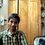# Where is the mistake

Hi guys.. I was just solving a problem related to parabolas and came across this situation.Everything I did seems right but there seems to be a mistake somewhere. Here goes the situation

There is a parabola (x-y)^2 = -48(x+y+4). So I figured that the axis must be x-y=0 and the tangent at the vertex must be x+y+4=0.So the vertex should be (-2,-2). Using the value of 'a' that is 8, I figured that the directrix should be 8 units away from the tangent at the vertex(this is because if you tilt the axes so that the axis of the parabola becomes the y axis,the distance between the tangent at the vertex and directrix must be 8.So even if I again tilt the axes back to the original position the distance between them remains the same) and found it to be x+y-4=0.So the point where the directrix intersects the axis is (2,2). Now comes the interesting part.When I found the distance between (2,2) and(-2.-2) it was not 8.I am shocked. I found the directrix taking the distance to be 8.But why isn't the distance not 8?5 years, 10 months ago

This discussion board is a place to discuss our Daily Challenges and the math and science related to those challenges. Explanations are more than just a solution — they should explain the steps and thinking strategies that you used to obtain the solution. Comments should further the discussion of math and science.

When posting on Brilliant:

• Use the emojis to react to an explanation, whether you're congratulating a job well done , or just really confused .
• Ask specific questions about the challenge or the steps in somebody's explanation. Well-posed questions can add a lot to the discussion, but posting "I don't understand!" doesn't help anyone.
• Try to contribute something new to the discussion, whether it is an extension, generalization or other idea related to the challenge.

MarkdownAppears as
*italics* or _italics_ italics
**bold** or __bold__ bold
- bulleted- list
• bulleted
• list
1. numbered2. list
1. numbered
2. list
Note: you must add a full line of space before and after lists for them to show up correctly
paragraph 1paragraph 2

paragraph 1

paragraph 2

[example link](https://brilliant.org)example link
> This is a quote
This is a quote
    # I indented these lines
# 4 spaces, and now they show
# up as a code block.

print "hello world"
# I indented these lines
# 4 spaces, and now they show
# up as a code block.

print "hello world"
MathAppears as
Remember to wrap math in $$ ... $$ or $ ... $ to ensure proper formatting.
2 \times 3 $2 \times 3$
2^{34} $2^{34}$
a_{i-1} $a_{i-1}$
\frac{2}{3} $\frac{2}{3}$
\sqrt{2} $\sqrt{2}$
\sum_{i=1}^3 $\sum_{i=1}^3$
\sin \theta $\sin \theta$
\boxed{123} $\boxed{123}$

Sort by:

The formulas that you used are only valid for parabolas of the form $x^2 = 4 ay$.When you use a different (orthogonal) basis like $(3x)^2 = 4 a ( 2y + 3 )$ then you have to consider how the diagram scales accordingly. In this example, note that the distance isn't just the value of $a$. Instead, we have to write it as $x^2 = 4 \times \left( \frac{2}{9} a \right) \times ( y + \frac{3}{2} )$, to conclude that the distance should be $\frac{2}{9} a$ instead.

In this case, you did a change of basis coordinates from $(x,y)$ to $(x-y, x+y + 4)$. This changed your distance by a factor of $\sqrt{2}$, and so the true distance would have been $\frac{8}{ \sqrt{2} } = 4 \sqrt{2}$. This agrees with the calculation that $\sqrt{ 4 ^2 + 4^2 } = 4 \sqrt{2}$.

Staff - 5 years, 10 months ago

Thank you.I understand my mistake

- 5 years, 10 months ago

Wo gawdd!! He understands his mistake!!!

- 1 year, 8 months ago